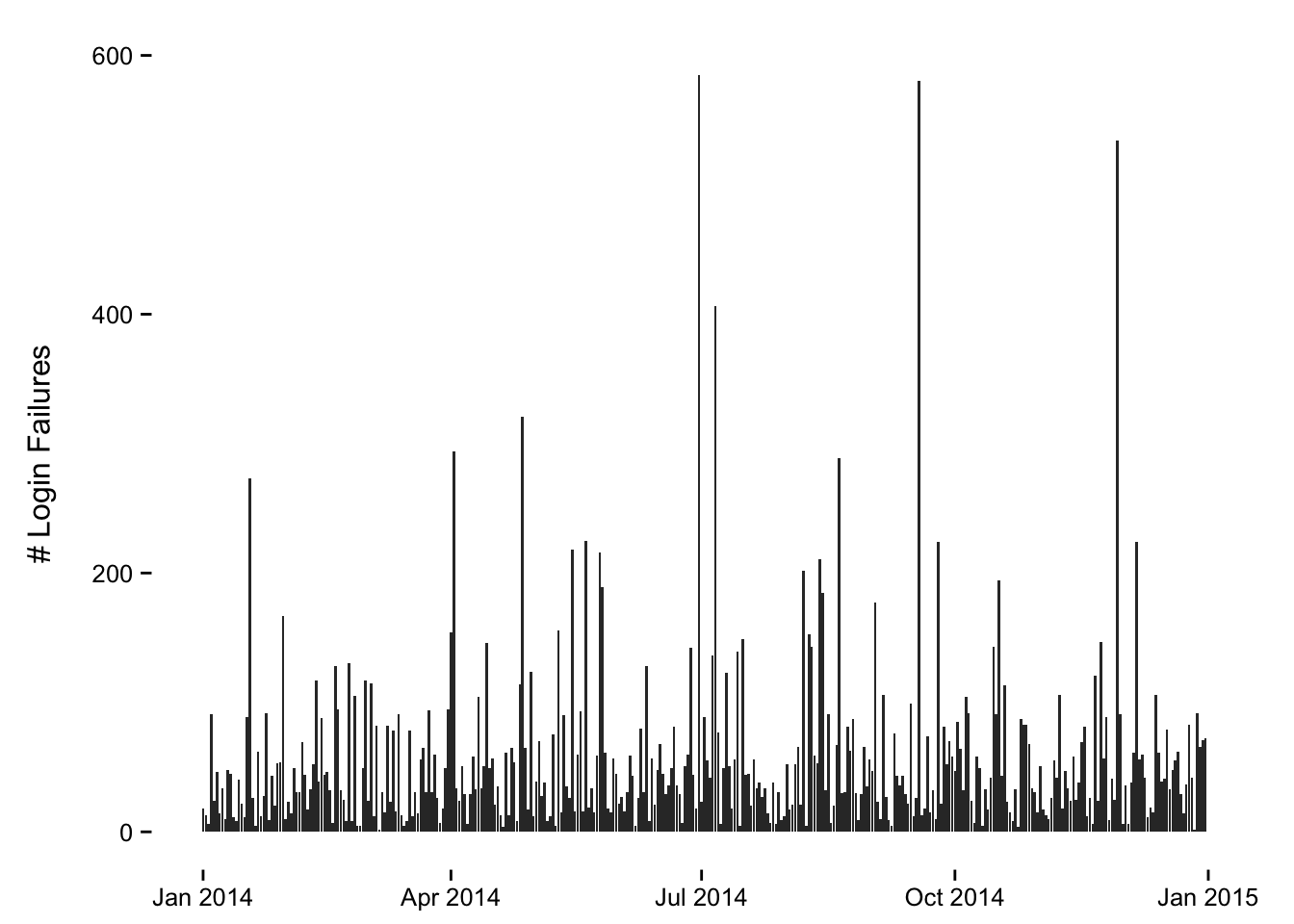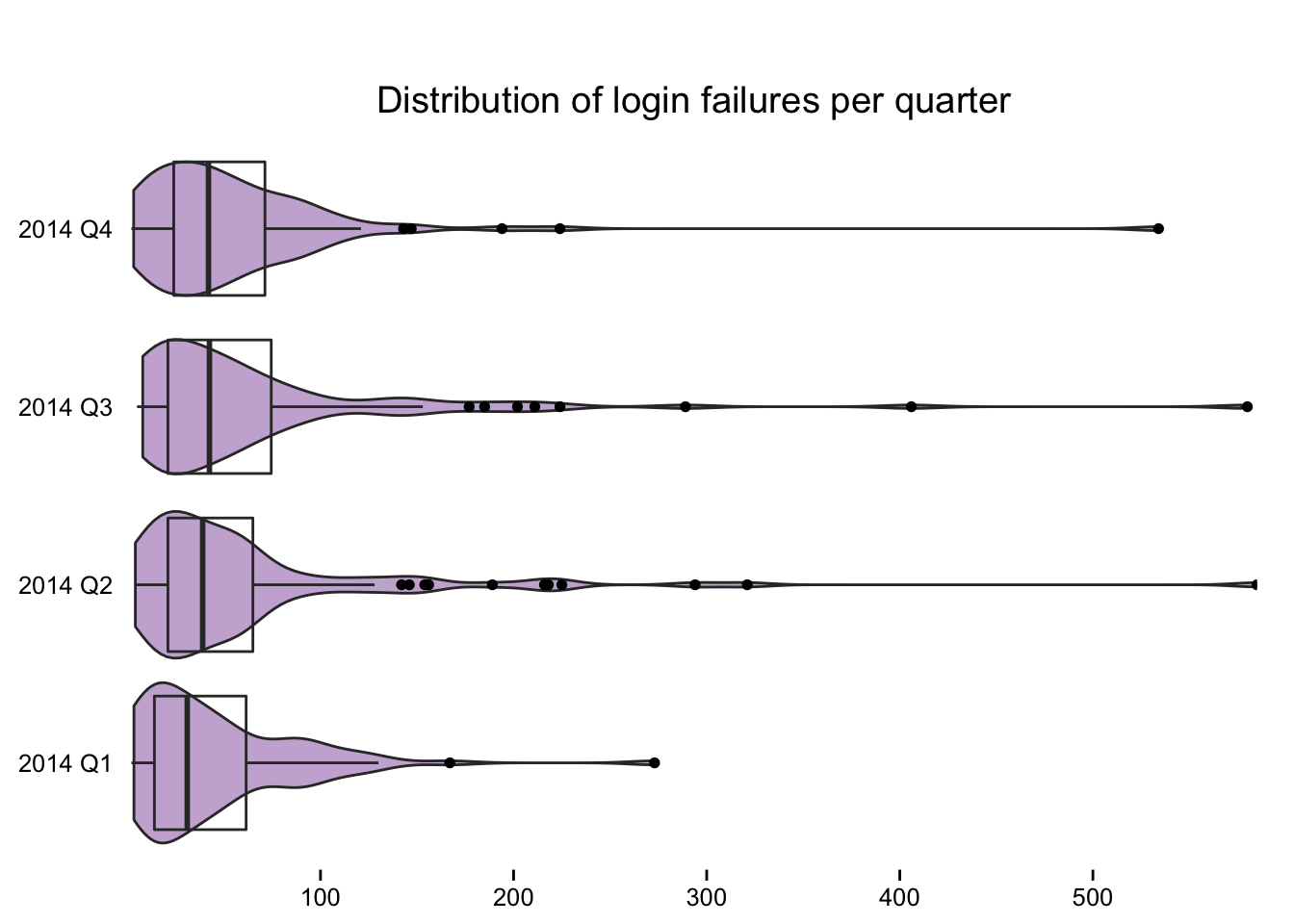R 101 - Aggregate By Quarter

By Bob Rudis (@hrbrmstr)
Tue 14 July 2015 | tags: blog, r, rstats, time series, r101, -- (permalink)

We were asked a question on how to (in R) aggregate quarterly data from what I believe was a daily time series. This is a pretty common task and there are many ways to do this in R, but we’ll focus on one method using the `zoo` and `dplyr` packages. Let’t get those imports out of the way:

```library(dplyr)
library(zoo)
library(ggplot2)
```

Now, we need some data. This could be from a database, log file or even Excel spreadsheet or CSV. Since we’re focusing on the aggregation and not the parsing, let’s generate some data, for daily failed logins in calendar year 2014:

```set.seed(1492)

yr_2014 <- seq(from=as.Date("2014-01-01"),
to=as.Date("2014-12-31"),
by="day")

failures=round(rlnorm(length(yr_2014)) *
sample(10:50, 1)), 0.5, 3)

## Observations: 365
## Variables:
## \$ date     (date) 2014-01-01, 2014-01-02, 2014-01-03, 2014-01-04, 2014...
## \$ failures (dbl) 18, 13, 6, 91, 24, 46, 14, 34, 10, 48, 45, 11, 8, 40,...
## \$ 0.5      (dbl) 0.5, 0.5, 0.5, 0.5, 0.5, 0.5, 0.5, 0.5, 0.5, 0.5, 0.5...
## \$ 3        (dbl) 3, 3, 3, 3, 3, 3, 3, 3, 3, 3, 3, 3, 3, 3, 3, 3, 3, 3,...
```

Using `set.seed` makes the pseudo-random draws via `rlnorm` repeatable on other systems. We can get a better look at that data:

```ggplot(logins, aes(x=date, y=failures)) +
geom_bar(stat="identity") +
labs(x=NULL, y="# Login Failures\n") +
theme_bw() +
theme(panel.grid=element_blank()) +
theme(panel.border=element_blank())
```We can then, summarize the number of failed logins by quarter using `as.yearqtr`:

```logins %>%
mutate(qtr=as.yearqtr(date)) %>%
count(qtr, wt=failures) -> total_failed_logins_by_qtr

## Source: local data frame [4 x 2]
##
##       qtr    n
## 1 2014 Q1 4091
## 2 2014 Q2 5915
## 3 2014 Q3 6141
## 4 2014 Q4 5229
```

NOTE: you can control the way those quarter labels look with the `format` parater to `as.yearqtr`:

`format`

character string specifying format. `"%C"`, `"%Y"`, `"%y"` and `"%q"`, if present, are replaced with the century, year, last two digits of the year, and quarter (i.e. a number between 1 and 4), respectively.

But you can also get more intra-quarter detail as well by looking at the distribution of failed logins:

```logins %>%
mutate(qtr=as.character(as.yearqtr(date))) %>%
ggplot() +
geom_violin(aes(x=qtr, y=failures), fill="#cab2d6") +
geom_boxplot(aes(x=qtr, y=failures), alpha=0) +
scale_y_continuous(expand=c(0, 0)) +
labs(x=NULL, y=NULL, title="\nDistribution of login failures per quarter") +
coord_flip() +
theme_bw() +
theme(panel.grid=element_blank()) +
theme(panel.border=element_blank()) +
theme(axis.ticks.y=element_blank())
```